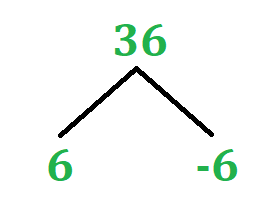GeeksforGeeks App
Open AppBrowser
Continue

## Related Articles

Real numbers are the numbers that include both rational and irrational numbers. Rational numbers such as integers (-2, 0, 1), fractions(1/2, 2.5), and irrational numbers such as √3, π(22/7), etc., are all real numbers. The definition of the nth root of a real number can be stated as:

For any two real numbers a and b, and any positive integer n, if an = b, then a is an nth root of b.

For Example:

34 = 81

Where,

a = 3, b = 81, and n = 4

That means that 3 is the fourth root of 81.

### How to write nth Roots?indicates an nth root.

For Example:Where,

n = Index

That means that we are taking the nth root of 144. One can take any value of n, like n = 2, 3, 5, etc. We will be taking that root of 100.

### Principal Root

Some numbers have more than one real nth root. For example, 36 has two roots, one is +6 and another is -6. In this case, the non-negative root is called the principal rootWhen no index is given the radical sign indicates the principal root. For example, if we takethen we are talking about its principal root which is 6.

### Examples

Example 1: If n = 5 and b = 32  then a = ?

Solution:

Given: a5 = 32

=>2 . 2 . 2 . 2 . 2 = 32

Since, 2 is the fifth root of 32

Therefore, a = 2

Example 2: n = 6 and b = 4096 then a = ?

Solution:

Given: a6 = 4096

=>To find the value of a, we will check which integer value is the sixth root of 4096

2 . 2 . 2 . 2 . 2 . 2 = 64

3 . 3 . 3 . 3 . 3 . 3 = 729

4 . 4 . 4 . 4 . 4 . 4 = 4096

Since, 4 is the sixth root of 4096

Therefore, a = 4

Example 3: If a = 18, b = 2, n  = 2, andthen find the value of c?

Solution:

Given:SInce the index value is same then the radicand is multipliedSince, 6 is the square root of 36 (62 = 36)

Therefore, c = 6

Example 4: If a = 5, b = 15, n = 3 andthen find the value of c?

Solution:

Given:SInce the index value is same then the radicand are dividedExample 5: Solve the following:Solution:

Given:1 . 1 . 1 = 1, 1 is the cube root of 1

2 . 2 . 2 = 8, 2 is the cube root of 8

3 . 3 . 3 = 27, 3 is the cube root of 27

4 . 4 . 4 = 64, 4 is the cube root of 64

c = 1 + 2 + 3 + 4

c = 10

My Personal Notes arrow_drop_up
Related Tutorials